The OpenFOAM Foundation
Boussinesq< Specie > Class Template Reference

Incompressible gas equation of state using the Boussinesq approximation for the density as a function of temperature only: More...

Inheritance diagram for Boussinesq< Specie >: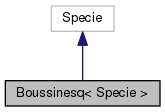[legend]
Collaboration diagram for Boussinesq< Specie >:[legend]

## Public Member Functions

Boussinesq (const Specie &sp, const scalar rho0, const scalar T0, const scalar beta)
Construct from components. More...

Boussinesq (const Boussinesq &sp)
Construct from Boussinesq. More...

Boussinesq (Istream &)
Construct from Istream. More...

Boussinesq (const dictionary &dict)
Construct from dictionary. More...

Boussinesq (const word &name, const Boussinesq &)
Construct as named copy. More...

autoPtr< Boussinesqclone () const
Construct and return a clone. More...

scalar rho (scalar p, scalar T) const
Return density [kg/m^3]. More...

scalar h (const scalar p, const scalar T) const
Return enthalpy departure [J/kmol]. More...

scalar cp (scalar p, scalar T) const
Return cp departure [J/(kmol K]. More...

scalar s (const scalar p, const scalar T) const
Return entropy [J/(kmol K)]. More...

scalar psi (scalar p, scalar T) const
Return compressibility rho/p [s^2/m^2]. More...

scalar Z (scalar p, scalar T) const
Return compression factor []. More...

scalar cpMcv (scalar p, scalar T) const
Return (cp - cv) [J/(kmol K]. More...

void write (Ostream &os) const
Write to Ostream. More...

void operator= (const Boussinesq &)

void operator+= (const Boussinesq &)

void operator-= (const Boussinesq &)

void operator*= (const scalar)

## Static Public Member Functions

static autoPtr< BoussinesqNew (Istream &is)

static autoPtr< BoussinesqNew (const dictionary &dict)

static word typeName ()
Return the instantiated type name. More...

## Static Public Attributes

static const bool incompressible = true
Is the equation of state is incompressible i.e. rho != f(p) More...

static const bool isochoric = false
Is the equation of state is isochoric i.e. rho = const. More...

## Friends

Boussinesq operator+ (const Boussinesq &, const Boussinesq &)

Boussinesq operator- (const Boussinesq &, const Boussinesq &)

Boussinesq operator* (const scalar s, const Boussinesq &)

Boussinesq operator== (const Boussinesq &, const Boussinesq &)

Ostreamoperator (Ostream &, const Boussinesq &)

## Detailed Description

### template<class Specie> class Foam::Boussinesq< Specie >

Incompressible gas equation of state using the Boussinesq approximation for the density as a function of temperature only:

```    rho = rho0*(1 - beta*(T - T0))
```
Source files

Definition at line 52 of file Boussinesq.H.

## Constructor & Destructor Documentation

 Boussinesq ( const Specie & sp, const scalar rho0, const scalar T0, const scalar beta )
inline

Construct from components.

Definition at line 33 of file BoussinesqI.H.

Referenced by Boussinesq< Specie >::Boussinesq().

Here is the caller graph for this function: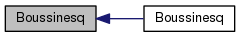Boussinesq ( const Boussinesq< Specie > & sp )
inline

Construct from Boussinesq.

Definition at line 46 of file BoussinesqI.H.

References Boussinesq< Specie >::Boussinesq(), and Foam::name().

Here is the call graph for this function: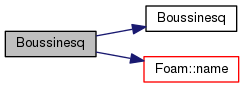Boussinesq ( Istream & is )

Construct from Istream.

Definition at line 32 of file Boussinesq.C.

References Boussinesq< Specie >::Boussinesq(), and IOstream::check().

Here is the call graph for this function: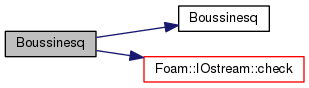Boussinesq ( const dictionary & dict )

Construct from dictionary.

Definition at line 49 of file Boussinesq.C.

 Boussinesq ( const word & name, const Boussinesq< Specie > & )
inline

Construct as named copy.

## Member Function Documentation

 Foam::autoPtr< Foam::Boussinesq< Specie > > clone ( ) const
inline

Construct and return a clone.

Definition at line 73 of file BoussinesqI.H.

References Boussinesq< Specie >::New().

Here is the call graph for this function: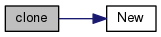Foam::autoPtr< Foam::Boussinesq< Specie > > New ( Istream & is )
inlinestatic

Definition at line 85 of file BoussinesqI.H.

Referenced by Boussinesq< Specie >::clone().

Here is the caller graph for this function: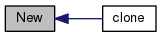Foam::autoPtr< Foam::Boussinesq< Specie > > New ( const dictionary & dict )
inlinestatic

Definition at line 99 of file BoussinesqI.H.

References dict, and Boussinesq< Specie >::rho().

Here is the call graph for this function: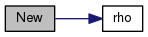static word typeName ( )
inlinestatic

Return the instantiated type name.

Definition at line 156 of file Boussinesq.H.

 Foam::scalar rho ( scalar p, scalar T ) const
inline

Return density [kg/m^3].

Definition at line 114 of file BoussinesqI.H.

Referenced by Boussinesq< Specie >::New().

Here is the caller graph for this function: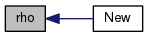Foam::scalar h ( const scalar p, const scalar T ) const
inline

Return enthalpy departure [J/kmol].

Definition at line 124 of file BoussinesqI.H.

 Foam::scalar cp ( scalar p, scalar T ) const
inline

Return cp departure [J/(kmol K].

Definition at line 131 of file BoussinesqI.H.

References Boussinesq< Specie >::s().

Here is the call graph for this function: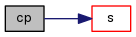Foam::scalar s ( const scalar p, const scalar T ) const
inline

Return entropy [J/(kmol K)].

Definition at line 139 of file BoussinesqI.H.

References Boussinesq< Specie >::psi().

Referenced by Boussinesq< Specie >::cp().

Here is the call graph for this function: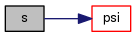Here is the caller graph for this function: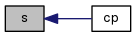Foam::scalar psi ( scalar p, scalar T ) const
inline

Return compressibility rho/p [s^2/m^2].

Definition at line 150 of file BoussinesqI.H.

References Boussinesq< Specie >::Z().

Referenced by Boussinesq< Specie >::s().

Here is the call graph for this function: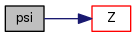Here is the caller graph for this function: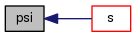Foam::scalar Z ( scalar p, scalar T ) const
inline

Return compression factor [].

Definition at line 161 of file BoussinesqI.H.

References Boussinesq< Specie >::cpMcv().

Referenced by Boussinesq< Specie >::psi().

Here is the call graph for this function: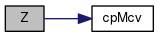Here is the caller graph for this function: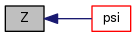Foam::scalar cpMcv ( scalar p, scalar T ) const
inline

Return (cp - cv) [J/(kmol K].

Definition at line 172 of file BoussinesqI.H.

References Foam::constant::thermodynamic::RR.

Referenced by Boussinesq< Specie >::Z().

Here is the caller graph for this function: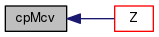void write ( Ostream & os ) const

Write to Ostream.

Definition at line 63 of file Boussinesq.C.

Here is the call graph for this function: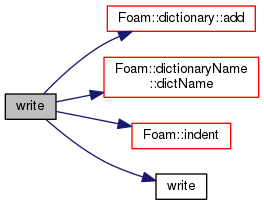void operator= ( const Boussinesq< Specie > & )
inline

Definition at line 185 of file BoussinesqI.H.

 void operator+= ( const Boussinesq< Specie > & )
inline

Definition at line 199 of file BoussinesqI.H.

 void operator-= ( const Boussinesq< Specie > & )
inline

Definition at line 216 of file BoussinesqI.H.

 void operator*= ( const scalar s )
inline

Definition at line 228 of file BoussinesqI.H.

References Foam::constant::physicoChemical::b, and s().

Here is the call graph for this function: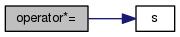## Friends And Related Function Documentation

 Boussinesq operator+ ( const Boussinesq< Specie > & , const Boussinesq< Specie > & )
friend
 Boussinesq operator- ( const Boussinesq< Specie > & , const Boussinesq< Specie > & )
friend
 Boussinesq operator* ( const scalar s, const Boussinesq< Specie > & )
friend
 Boussinesq operator== ( const Boussinesq< Specie > & , const Boussinesq< Specie > & )
friend
 Ostream& operator ( Ostream & , const Boussinesq< Specie > & )
friend

## Member Data Documentation

 const bool incompressible = true
static

Is the equation of state is incompressible i.e. rho != f(p)

Definition at line 167 of file Boussinesq.H.

 const bool isochoric = false
static

Is the equation of state is isochoric i.e. rho = const.

Definition at line 170 of file Boussinesq.H.

The documentation for this class was generated from the following files:
• src/thermophysicalModels/specie/equationOfState/Boussinesq/Boussinesq.H
• src/thermophysicalModels/specie/equationOfState/Boussinesq/Boussinesq.C
• src/thermophysicalModels/specie/equationOfState/Boussinesq/BoussinesqI.H